# Generate New MNIST Digits Using Generative Adversarial Neural Networks (GAN)

Posted 2018-11-11

### Goal:¶

• Build a GAN that generates new handwritten digits [0, 1, 2, 3, 4, 5, 6, 7, 8, 9].

• Implementation uses an adversarial competition between a Generator and Discriminator where the goal of the Generator is to create an image from scratch that fools the Discriminator into believing it's a 'real' image versus a fake generated one.

• Peer a bit under the hood into the effects of the assumptions we inherently make in building this GAN network.

• This is a reproduction (with additional expansions of my own) from the AIND Deep learning course with MNIST dataset

### High Level Thoughts / Findings:¶

Something interesting about training GAN's is that unlike other classification tasks, the losses are just a distant proxy of our real goal (see Fig. 1 and Fig. 2) even though we have the ground truth labels (1 = real, 0 = fake). Our real goal is for Generator G to learn how to produce digits that look like real data, but the way the model is designed, its goal is to fool the Discriminator D. While it seems similar at first glance, the nuance is that D itself doesn't have a 'ground truth' idea of what a real digit should look like. Imagine an extreme example where G ends up confusing D and making it dumber and dumber (ie. increasing both false positives and false negatives). G 'wins' but G isn't necessarily much better at creating realistic looking digits (to humans).

In part this is because both D and G are evolving and learning from scratch at the same time. D & G does have good analogy to 'Cops and Robbers' but it's just in this case, the cops do not know what's the law to start with, so its view of the law may become fuzzy over time.

Future work could investigate a D that's pre-trained to already recognize digits well.

### Implicity Assumptions / Hyperparameters:¶

To get a real feel of the model I like enumerating explicitly the assumptions and hyperparameters baked into it. Theoretically these can be relaxed or tuned.

• Data
• Fixed input dimensions 28x28 pixels flattened to 1D vector
• Graysale digits cleaned and centered
• Normalization of features from -1->1
• Model
• Initial input size for Generator
• Initialization of random input for Generator
• Initialization of weights and biases
• # of layers and units
• Activation function - leaky RELU
• Training
• Optimizers
• Learning rate
• Batch size
• Regularization of loss function
• Loss function for descriminator where false positives and false negatives are treated symmetrically
• Ground truth is known to model
• Randomize training sequence during training
In :
import warnings
warnings.filterwarnings('ignore')

In :
# System import
import math
import pickle as pkl

# 3rd party libraries
import matplotlib.pyplot as plt
import numpy as np
from sklearn.utils import shuffle
import tensorflow as tf
from tqdm import tqdm_notebook as tqdm

# My own custom package library
from datasets import MNIST

%matplotlib inline


## I. Data¶

### Import and Preprocess MNIST dataset¶

In :
X_train, Y_train, X_test, Y_test = MNIST.load_data()
MNIST.show_sample_data(X_train, Y_train, 4, 4)

Out: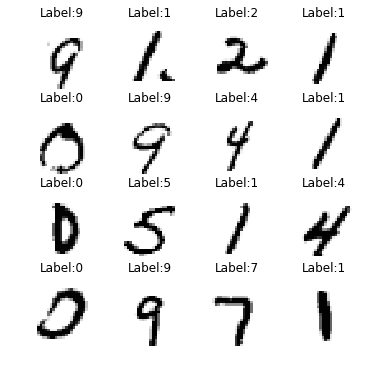In :
X_train, Y_train = MNIST.preprocess(X_train, Y_train,
flatten=True, one_hot=True)
X_train = MNIST.rescale(X_train, neg_one_to_one=True)
X_train.shape, Y_train.shape

Out:
((60000, 784), (60000, 10))

## II. Building Model¶### GanGame class¶

In :
class GanGame:
"""An adversarial competition between Generator and Discriminator where
the interaction is for the Generator to generate a new image and fool
the Discriminator into believing that it is in fact a 'rea' image.
"""
def __init__(self, smooth=0.1, data_dim=784,
checkpoint_dir='./checkpoints/'):
"""Constructor.

Params:
smooth (float): correction for cross entropy even when correct,
loss slightly > 0
data_dim (int): dimensions of data for interface between
generator and discriminator
checkpoint_dir (string): directory to save checkpoint files
"""
self.__data_dim = data_dim
self.__smooth = smooth
self.__checkpoint_dir = checkpoint_dir

def get_smooth(self):
return self.__smooth

def get_data_dim(self):
return self.__data_dim

def set_g_loss(self, gen_logits):
"""Set generator loss function. 'Correct' is if discriminator
classified as 1, ie. the discriminator is fooled by the generator.

Params:
gen_logits (ndarray): output logits from generator
"""
self.g_loss = tf.reduce_mean(
tf.nn.sigmoid_cross_entropy_with_logits(
logits=gen_logits,
labels=tf.ones_like(gen_logits)
* (1 - self.__smooth)))

def set_d_loss(self, real_logits, gen_logits, real_data_penalty=1):
"""Set discriminator loss function. Defined in 2 parts. For real
dataset input, 'correct' is if discriminator classified as 1 (ie.
came from the real dataset).  Penalty can be applied to weight real
data losses more heavily vs. fake data losses.

For generator input, 'correct' is if discriminator classified as 0
(ie. came from the generator)

Params:
real_logits (ndarray): logits from real dataset
gen_logits (ndarray): logits from generator
real_data_penalty (int): power exponent on loss from real data
"""
d_real_loss = tf.reduce_mean(
tf.nn.sigmoid_cross_entropy_with_logits(
logits=real_logits,
labels=tf.ones_like(real_logits)
* (1 - self.__smooth)))
d_gen_loss = tf.reduce_mean(
tf.nn.sigmoid_cross_entropy_with_logits(
logits=gen_logits,
labels=tf.zeros_like(gen_logits)))
self.d_loss = d_real_loss**real_data_penalty + d_gen_loss

def get_trainable_vars(self):
"""Get set of trainable variables from the TF graph. """
self.g_vars = [_ for _ in tf.trainable_variables()
if _.name.startswith('generator')]
self.d_vars = [_ for _ in tf.trainable_variables()
if _.name.startswith('discriminator')]

def set_optimizers(self, learning_rate=0.002):
"""Set loss optimizers for discriminator and generator.

Params:
learning_rate (float): learning rate of the optimizer
"""
.minimize(self.d_loss, var_list=self.d_vars)
.minimize(self.g_loss, var_list=self.g_vars)

def train(self, X_train, d_real, d_gen, gen, epochs=100, batch_size=100,
verbose=False, save_weight_epoch=None, data_shuffle=True,
ckpt_filename='model.ckpt'):
"""Training the discriminator and generator.

Params:
X_train (ndarray): training data
d_real (Discriminator): discriminator for real dataset
d_gen (Discriminator): discriminator for generator dataset
gen (Generator):
epochs (int): number of epochs to train
batch_size (int): mini batch size within each epoch
verbose (bool): show discriminator and generator losses at each
epoch
save_weight_epoch (bool): save model weights every x epoch
specified
data_shuffle (bool): shuffle training data for every epoch
ckpt_filename (string): checkpoint filename
"""
losses = []
gan_training_samples = []
gan_training_samples_logits = []
d_training_labels = []
d_real_training_labels = []
saver = tf.train.Saver(var_list=self.g_vars+self.d_vars,
max_to_keep=100)

with tf.Session() as sess:
sess.run(tf.global_variables_initializer())

for e in tqdm(range(epochs)):
if data_shuffle:
X_train = shuffle(X_train)
batched_data = MNIST.batch_data(X_train, None, batch_size)
for i in range(math.ceil(len(X_train) / batch_size)):
batch_X_train = next(batched_data)
batch_z = np.random.uniform(-1, 1,
size=(len(batch_X_train),
gen.input_size))
# Train batch
_ = sess.run(self.d_train_opt,
feed_dict={d_real.inputs: batch_X_train,
gen.inputs: batch_z})
_ = sess.run(self.g_train_opt,
feed_dict={gen.inputs: batch_z})

# Losses for epoch
train_d_loss = sess.run(self.d_loss,
feed_dict={
d_real.inputs: batch_X_train,
gen.inputs: batch_z})
train_g_loss = sess.run(self.g_loss,
feed_dict={gen.inputs: batch_z})
losses.append((train_d_loss, train_g_loss))

# Variables to save
if save_weight_epoch is not None \
and e % save_weight_epoch == 0:
saver.save(sess, self.__checkpoint_dir+ckpt_filename,
write_meta_graph=False, global_step=e)

gen_samples_logits, gen_samples = \
sess.run(gen.build_NN(reuse=True),
feed_dict={gen.inputs: batch_z})
gan_training_samples.append(gen_samples)
gan_training_samples_logits.append(gen_samples_logits)

d_labels_logits, d_labels = \
sess.run(d_real.build_NN(d_real.inputs, reuse=True),
feed_dict={d_real.inputs: gen_samples})
d_training_labels.append(d_labels)

d_real_labels_logits, d_real_labels = \
sess.run(d_real.build_NN(d_real.inputs, reuse=True),
feed_dict={d_real.inputs: batch_X_train})
d_real_training_labels.append(d_real_labels)

if verbose:
if e == 0:
print("epoch \td_loss \tg_loss")
print("{:d}\t{:.4f}\t{:.4f}".format(e+1, train_d_loss,
train_g_loss))

# Cleanup and dump to disk
self.losses = np.array(losses)
gan_training_samples = np.array(gan_training_samples)
d_training_labels = np.array(d_training_labels)
d_real_training_labels = np.array(d_real_training_labels)

if save_weight_epoch is not None:
with open('gan_training_samples.pkl', 'wb') as f:
pkl.dump(gan_training_samples, f)
f.close()
with open('gan_training_samples_logits.pkl', 'wb') as f:
pkl.dump(gan_training_samples_logits, f)
f.close()
with open('d_training_labels.pkl', 'wb') as f:
pkl.dump(d_training_labels, f)
f.close()
with open('d_real_training_labels.pkl', 'wb') as f:
pkl.dump(d_real_training_labels, f)
f.close()
with open('gan_init.pkl', 'wb') as f:
pkl.dump(batch_z, f)
f.close()

def generate_samples(self, gen, samples):
"""Based on the final training model, generate new samples.

Params:
gen (Generator): generator
samples (int): number of samples to generate

Returns:
(ndarray) samples
"""
saver = tf.train.Saver(var_list=self.g_vars)
with tf.Session() as sess:
saver.restore(sess,
tf.train.latest_checkpoint(self.__checkpoint_dir))
sample_z = np.random.uniform(-1, 1,
size=(samples, gen.input_size))
gen_samples_logits, gen_samples = \
sess.run(gen.build_NN(reuse=True),
feed_dict={gen.inputs: sample_z})
sess.close()
return gen_samples

def generate_samples_labels(self, gen, samples, d_real):
"""Wrapper for self.generate_samples to include labels from
discriminator.

Params:
See self.generate_samples
d_real (Discriminator): discriminator

Returns:
(ndarray), (ndarray) generated samples and labels
"""
gen_samples = self.generate_samples(gen, samples)

saver = tf.train.Saver(var_list=self.d_vars)
with tf.Session() as sess:
saver.restore(sess,
tf.train.latest_checkpoint(self.__checkpoint_dir))
d_labels_logits, d_labels = \
sess.run(d_real.build_NN(d_real.inputs, reuse=True),
feed_dict={d_real.inputs: gen_samples})
sess.close()
return gen_samples, d_labels



### Generator Class¶In :
class Generator:
"""Generator to create images."""
def __init__(self, game, alpha=0.01, input_size=100, h1_size=128):
"""Constructor.

Params:
game (GanGame): GAN game object
alpha (float): correction for leaky RELU
input_size (int): size of random initialized image
h1_size (int): hidden layer1 units
"""
self.alpha = alpha
self.input_size = input_size
self.h1_size = h1_size
self.output_dim = game.get_data_dim()
self.inputs = tf.placeholder(tf.float32, [None, input_size])

def build_NN(self, reuse=False):
"""Buid the neural network architecture. All generator instances
will interact with TF graphs in the scope of 'generator'

Params:
resuse (bool): Reuse the weights before if True, ie. access the
same variables in TF
"""
with tf.variable_scope('generator', reuse=reuse):
h1 = tf.layers.dense(self.inputs, self.h1_size, activation=None)
h1 = tf.maximum(self.alpha * h1, h1, name='h1_out')
yhat_logits = tf.layers.dense(h1, self.output_dim,
activation=None)
yhat = tf.tanh(yhat_logits, name='h2_out')
return yhat_logits, yhat


### Discriminator class¶In :
class Discriminator:
"""Discriminator to classify if image came from real dataset
or generator.
"""
def __init__(self, game, alpha=0.01, h1_size=128, output_dim=1):
"""Constructor.

Params:
game (GanGame): GAN game object
alpha (float): correction for leaky RELU
h1_size (int): hidden layer1 units
output_dim (int): output dimensions of discriminator
"""
self.alpha = alpha
self.h1_size = h1_size
self.output_dim = output_dim
self.inputs = tf.placeholder(tf.float32, [None, game.get_data_dim()])

def build_NN(self, inputs, reuse=False):
""" Build the neural network architecture. All discriminator instances
will interact with TF graphs in the scope of 'discriminators'. Reusing
enables accessing the same model weights in TF across different
discriminators instances.

Params:
inputs (ndarray): input data to the discriminator. Can be concrete
data or TF output
reuse (bool): Reuse the same TF variable weights if True
"""
#
with tf.variable_scope('discriminator', reuse=reuse):
h1 = tf.layers.dense(inputs, self.h1_size, activation=None)
h1 = tf.maximum(self.alpha * h1, h1, name='h1_out')
yhat_logits = tf.layers.dense(h1, self.output_dim,
activation=None)
yhat = tf.sigmoid(yhat_logits, name='h2_out')
return yhat_logits, yhat


## III. Main Loop¶

In :
# Create game
tf.reset_default_graph()
game = GanGame()

# Create generator
gen = Generator(game)
g_yhat_logits, g_yhat = gen.build_NN()

# Create 2 discriminators instance to handle 2 different inputs but both
# point to the same TF model (weights, biases)
d_real = Discriminator(game)
d_real_yhat_logit, d_real_yhat = d_real.build_NN(d_real.inputs, reuse=False)
d_gen = Discriminator(game)
d_gen_yhat_logit, d_gen_yhat = d_gen.build_NN(g_yhat, reuse=True)

# Set losses and optimizers
game.set_d_loss(d_real_yhat_logit, d_gen_yhat_logit, real_data_penalty=2)
game.set_g_loss(d_gen_yhat_logit)
game.get_trainable_vars()
game.set_optimizers()

# Run the TF graph and train the model
game.train(X_train, d_real, d_gen, gen, verbose=False,
epochs=100, save_weight_epoch=10)


## IV. Analysis¶

### Visualize Performance Over Epochs¶

In :
fig, ax = plt.subplots(figsize=(10,7))
plt.plot(game.losses[:,0], label='Discriminator')
plt.plot(game.losses[:,1], label='Generator')
plt.legend()
ax.set_xlabel('Fig 1. Losses for D and G at each epoch')

Out:
<matplotlib.text.Text at 0x1a355864e0>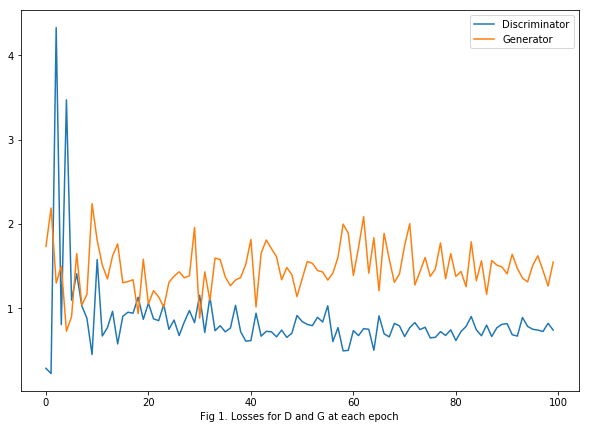### Discriminator - False Positives and Negatives of a Mini-Batch Over Time¶

In :
with open('d_real_training_labels.pkl', 'rb') as f:
f.close()
with open('d_training_labels.pkl', 'rb') as f:
f.close()

In :
fig, ax = plt.subplots(2, len(d_training_labels), figsize=(15,8), sharey=True)
for idx, gen_box, real_box in zip(range(len(d_training_labels)),
np.squeeze(d_training_labels),
np.squeeze(d_real_training_labels)):
ax[0, idx].boxplot(gen_box)
ax[1, idx].boxplot(real_box)
ax[0, idx].set_xticklabels([])
ax[1, idx].set_xticklabels([idx+1])

ax[0,0].set_ylabel('Discr. Classify - generated data set')
ax[1,0].set_ylabel('Discr. Classify - real data set')
fig.suptitle('Fig 2. Classification Spread of Real vs Generator Over Training')

Out:
<matplotlib.text.Text at 0x1a2fde7dd8>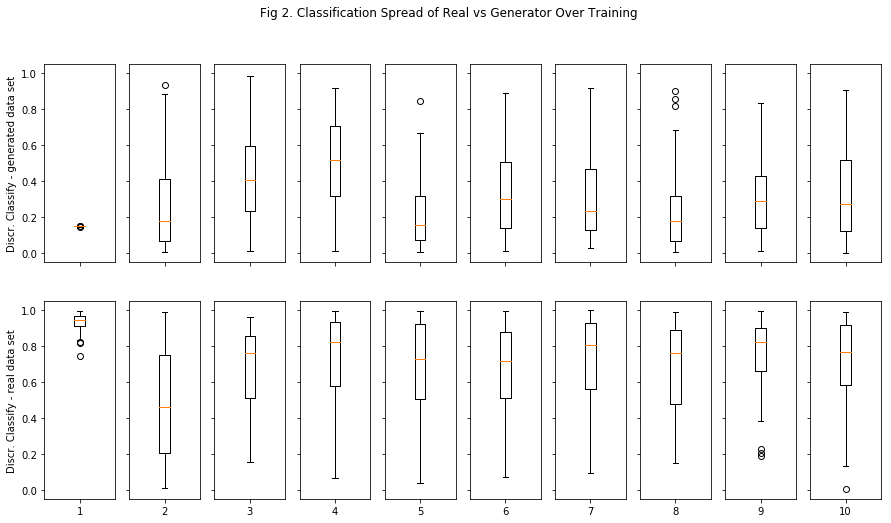### Visualize Initialized Input for Generator¶

In :
with open('gan_init.pkl', 'rb') as f:
f.close()

fig = MNIST.show_sample_grid(gan_init, 4, 4, reshape_to_2D=True)
fig.suptitle('Fig 3. Random Uniform from -1 to 1')

Out:
<matplotlib.text.Text at 0x1a3000f898>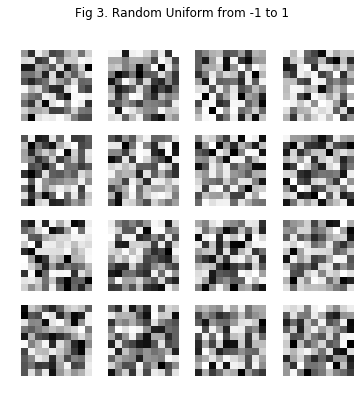### Generator Samples and Discriminator Label During Training Epochs¶

#### Pre-application of sigmoid on the generator logits¶

In :
with open('gan_training_samples_logits.pkl', 'rb') as f:
f.close()

for epoch_samples_logit, epoch_labels in zip(gan_training_samples_logits,
d_training_labels):
MNIST.show_sample_grid(epoch_samples_logit,
Y_data=epoch_labels,
rows=1,
cols=8,
reshape_to_2D=True,
skip_interval=True,
img_scale=10)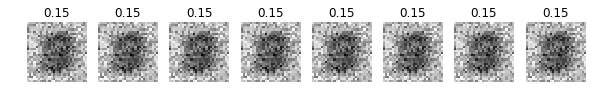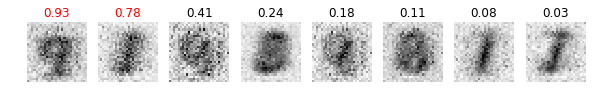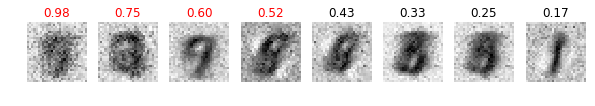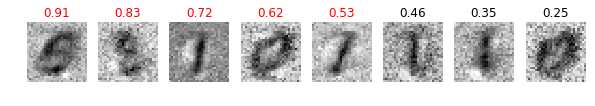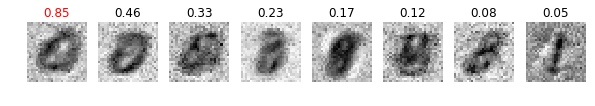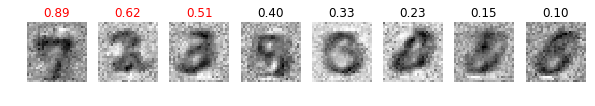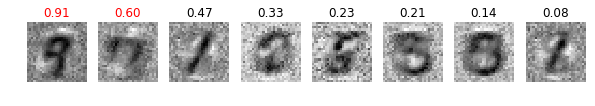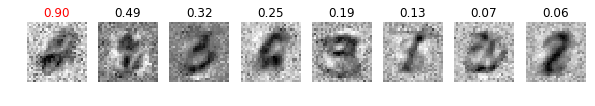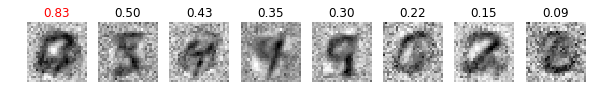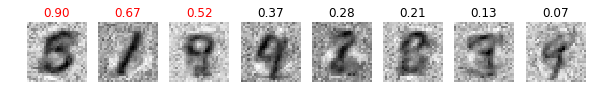#### Post-application of sigmoid¶

In :
with open('gan_training_samples.pkl', 'rb') as f:
f.close()

for epoch_samples, epoch_labels in zip(gan_training_samples,
d_training_labels):
MNIST.show_sample_grid(epoch_samples,
Y_data=epoch_labels,
rows=1,
cols=8,
reshape_to_2D=True,
skip_interval=True,
img_scale=10)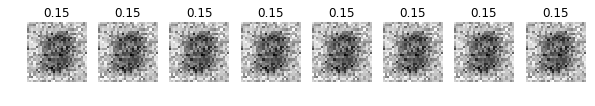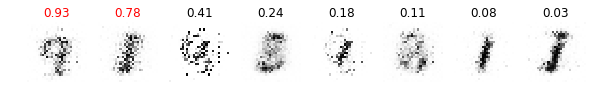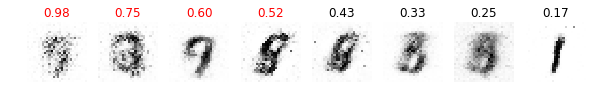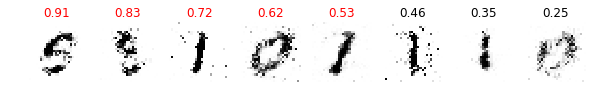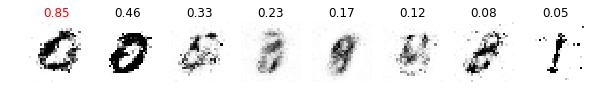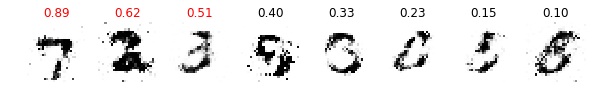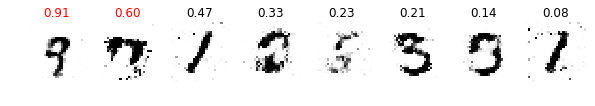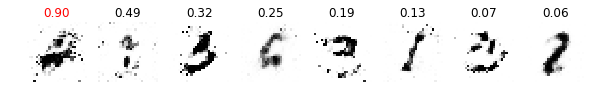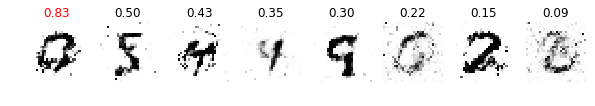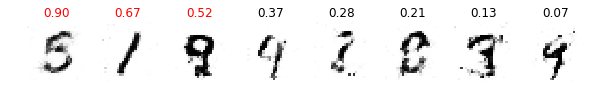### Generate New Samples from Final Trained Model¶

In :
g_samples, d_labels = game.generate_samples_labels(gen, 36, d_real)
fig = MNIST.show_sample_grid(X_data=g_samples, Y_data=d_labels,
rows=6, cols=6, reshape_to_2D=True)
fig.suptitle('Fig 6. Generated Digits and Classifications')

INFO:tensorflow:Restoring parameters from ./checkpoints/model.ckpt-90
INFO:tensorflow:Restoring parameters from ./checkpoints/model.ckpt-90

Out:
<matplotlib.text.Text at 0x1a31527400>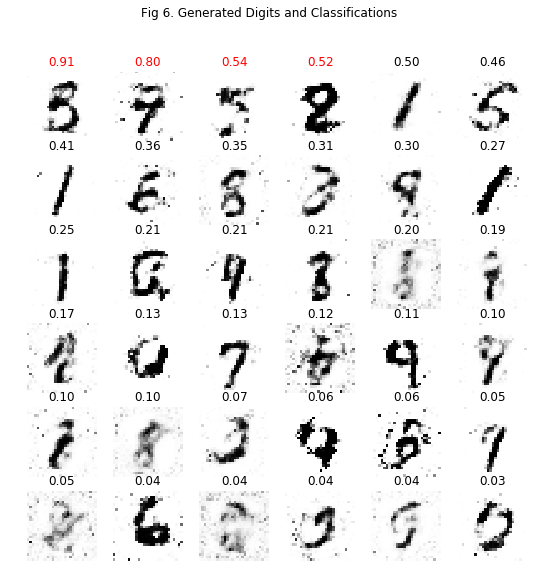In [ ]: